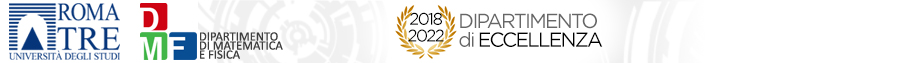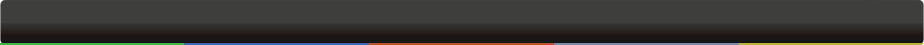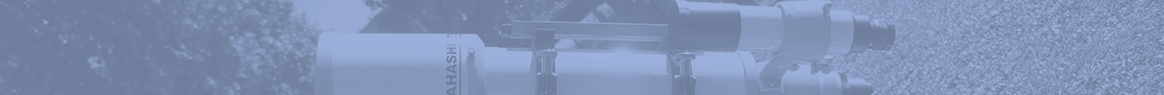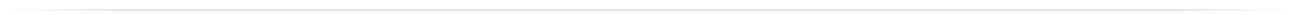# Seminari del Dipartimento

 GeometriaReconstructing curves from their Hodge classesMaria Gioia Cifani 25-02-2021 - 15:00modalità telematica (telematic form) Let S be a smooth algebraic surface in $P^3$. A curve C in S has a cohomology class [C] in $H^1( Ω^1_S)$. The Hodge class of C is the equivalence class of [C] in the quotient modulo the subspace generated by the class of a plane section of S. Recently, Movasati and Sertoz pose several interesting questions about the reconstruction of C from its Hodge class. In particular they give the notion of a perfect class: the Hodge class of a curve C is perfect if its annihilator is a sum of ideals of curves C whose Hodge class is a nonzero rational multiple of that of C. I will report on a joint work with Gian Pietro Pirola and Enrico Schlensiger, in which we give an answer to some of these questions: we show that the Hodge class of a smooth rational quartic on a surface of degree 4 is not perfect, and that the Hodge class of an arithmetically Cohen-Macaulay curve is always perfect.org: Bolognese Barbara